# 早下班系列：轻松玩转Excel行列转换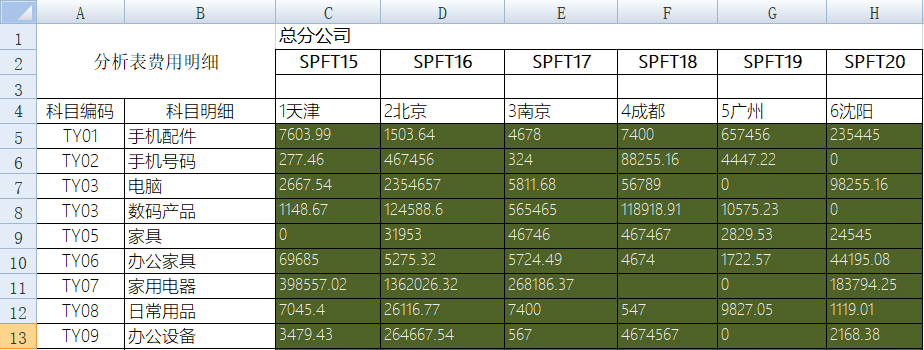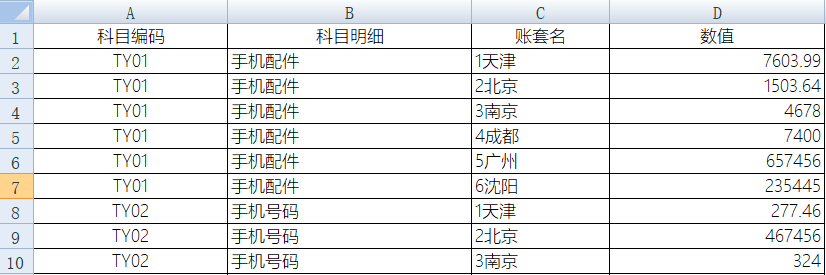Excel也可以通过数据透视表支持行列转换功能，效果如下图：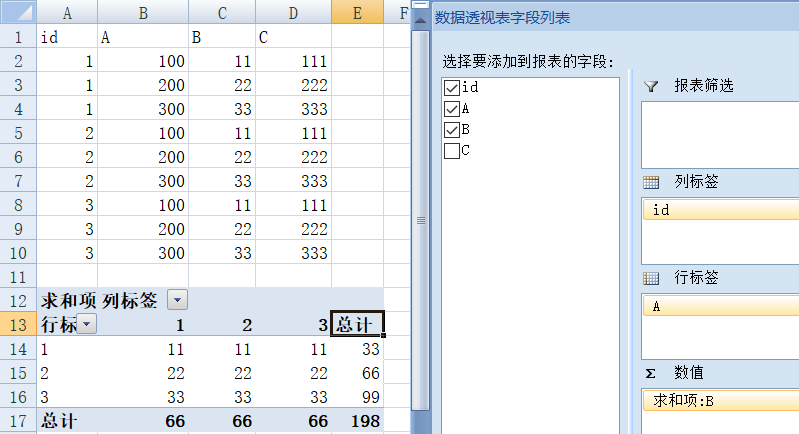1. 加载excel文件，装载需要的sheet工作表。
2. 读取账套名所在的行，将其转换成字符串数组。
3. 读取科目编码所在列，将其转换成字符串数组。
4. 按科目编码分组，与账套名数组构造一张表。
5. 根据账套名对应的数据，遍历所有的明细值填充到相应的表中。
6. 这样就构造出对应的明细表来。

import pandas as pd
import numpy as np

cols = df.columns.values.tolist() #获取数据头信息

#移去前两列，只保留需要行列转换的列

cols.remove('科目编码')

cols.remove('科目明细')

#构造一个list.

frames=[]

for col in cols:

df1 = df.pivot_table(index = ['科目编码','科目明细'], values = [col])

df1.rename(columns={col: '数值'}, inplace=True)

df1=col

#转换后的数据追加到frames中.

frames.append(df1)

# concat将相同字段的表首尾相接

result=pd.concat(frames)

result.rename(columns={3: '帐套名'}, inplace=True)

result.to_excel('D:\\excel\\pandas_n.xlsx', sheet_name='科目明细')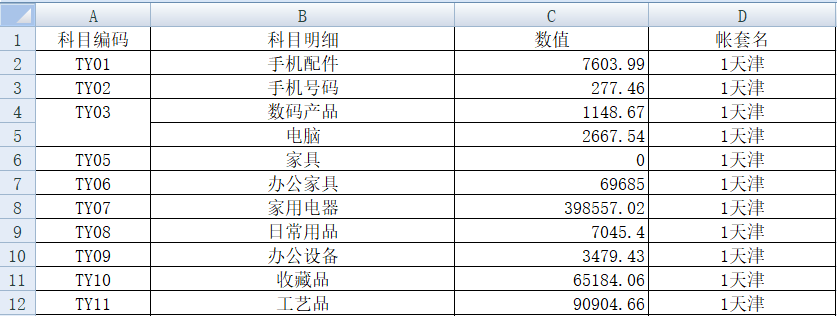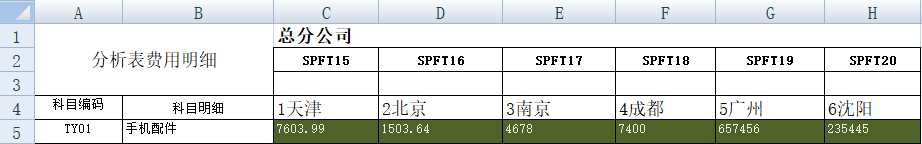A B 1 =file("D:/excel/明细.xlsx").importxls@t(;1,3:40) //读入excel文件 2 >A1.delete(A1.select(_1=="科目编码")) //清除首列为"科目编码"所在的行 3 >A1.rename(_1:科目编码,_2:科目明细) //更换列1名称为科目编码，列2名称为科目明细 4 =A1.fname().to(3,).concat(",") //将从第三列的列名连成字符串，用,分开 5 =A1.pivot@r(科目编码,科目明细;账套名,数值;\${A4}) //用pivot函数进行行列转换 6 =file("D:/excel/明细2.xlsx").exportxls@t(A5;"科目明细") //将整理好的数据另存储为xlsx文件

A1：加载excel文件工作表1，提取指定范围的数据(从3行到40行)，其中选项@ t表示首行为标题，载入数据,生成表格如下：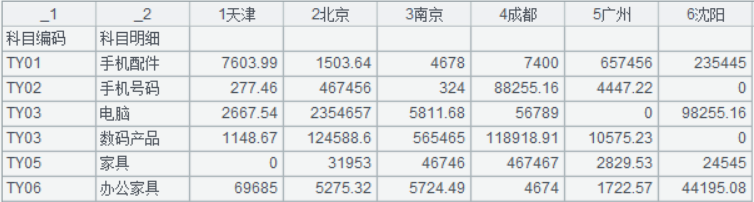A2：删除非数据行A3：更换列名称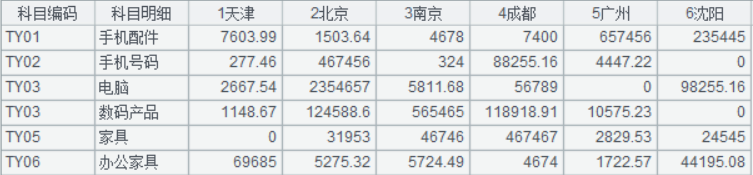A4：把从第三列开始的列名称连成字符串，用“,”分开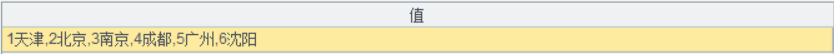A5：pivot函数将行列数据进行转换，把A4中对应的列数据置放到“数值”列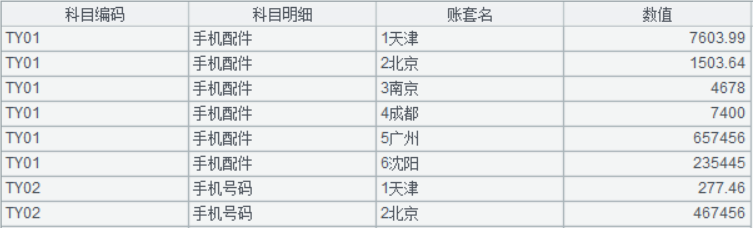A6：将整理好的数据另存储为xls文件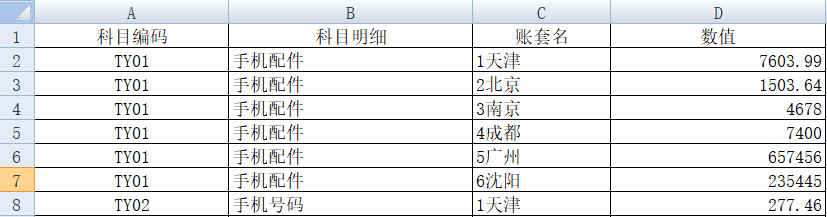### 2.名称更改：

python对于需要转换列的名称不能更改,如cols=’天津’，此时python找不到修改前的关键字，“哪个朋友挖的坑，别以为我发现不了”，欺负大爷眼花，给报个异常行不？

### 3.标题空值问题：

Python读取excel表中的转换行标题时，前面两列为空(对应原来的excel中的“科目编码，科目明细”)，此时标题cols中的空值就没有了,这个“坑”有点隐蔽啊，我真没有发现,把其中的两列弄丢了，真有点丢脸 ;